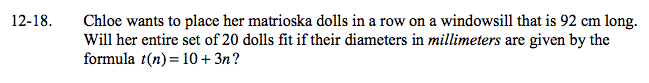### Home > A2C > Chapter 12 > Lesson 12.1.1 > Problem12-18

12-18.

Chloe wants to place her matrioska dolls in a row on a windowsill that is 92 cm long Will her entire set of 20 dolls fit if their diameters in millimeters are given by the formula t(n) = 10 + 3n? Homework Help ✎Graph the series from t(1) to t(20).

Rearrange the graph into a single rectangle and find the area.

Is the total less than 92 cm?

Pay careful addition to units. How many millimeters are in a centimeter?